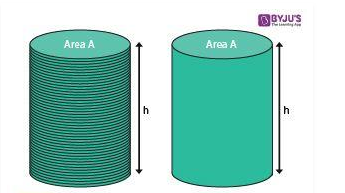### Cylinder

Cylinder is one of the basic shapes, in Mathematics, which has two parallel circular bases at a distance (called height of cylinder). LPG gas-cylinder is one of the real-life examples. It is a three-dimensional shape having surface area and volume. The total area of cylinder is equal to the sum of its curved surface area and area of the two circular bases.

Here we will learn about its definition, formulas, properties of cylinder and will solve some examples based on them. Apart from this figure, we have concepts of Sphere, Cone, Cuboid, Cube, etc. which we learn in Solid Geometry.

## Definition of Cylinder

A cylinder is a three-dimensional solid that holds two parallel bases joined by a curved surface, at a fixed distance. These bases are normally circular in shape and the center of the two bases are joined by a line segment, which is called as the axis. The perpendicular distance between the bases is the height, “h” and the distance from the axis to the outer surface is the radius “r” of the cylinder.### Cylinder Formulas

The cylinder has three major quantities, based on which we have the formulas.

• Lateral Surface Area or curved surface area
• Total Surface Area
• Volume

Let us see, their formulas now.

Curved Surface Area: The area of the curved surface of the cylinder which is contained between the two parallel circular bases. It is also stated as a lateral surface area. The formula for it is given by:

 Curved Surface Area = 2πrh square units

Total Surface Area: The total surface area of a cylinder is the sum of curved surafce area and the area of two circular bases.
TSA = Curved surface + Area of Circular bases
TSA = 2πrh + 2πr2
We can see, from the above expression, 2πr is common. Therefore,

 Total surface area, A = 2πr(r+h) square units

Volume: The volume of the cylinder is the space occupied by it in any three-dimensional plane. The amount of water that could be immersed in a cylinder is described by its volume. The formula for the colume is given by:

 Volume of the Cylinder, V = πr2h cubic units

Where ‘h’ is the height and ‘r’ is the radius.
Also, read: Area Of Hollow Cylinder

### Properties of Cylinder

The basic properties of the cylinder are;

• The bases are always congruent and parallel.
• If axis forms a right angle with the bases, which are exactly over each other, then it is called as “Right Cylinder”.
• It is similar to the prism since it has the same cross-section everywhere.
• If the bases are not exactly over each other but sideways, and the axis does not produce the right angle to the bases, then it is called “Oblique Cylinder”.
• If the bases are circular in shape, then it is called a right circular cylinder.
• If the bases are in an elliptical shape, then it is called an “Elliptical Cylinder”.

### Cylinder Examples

Question: Find the total surface area of the cylinder, whose radius is 5cm and height is 10cm?

Solution: We know, from the formula,

Total surface area of a cylinder, A = 2πr(r+h) square units

Therefore, A = 2π × 5(5 + 10) = 2π × 5(15) = 2π × 75 = 150 × 3.14 = 471 cm2

Question: What is the volume of a cylindrical shape water container, whose has the height of 7cm and diameter of 10cm?

Solution: Given,

Diameter of the container = 10cm

Thus, the radius of the container = 10/2 = 5cm

Height of the container = 7cm

As we know, from the formula,

Volume of a cylinder = πr2h cubic units.

Therefore, volume of the given container, V = π × 5× 7

V = π × 25 × 7 = (22/7) × 25 × 7 = 22 × 25

V = 550 cm3